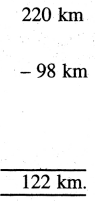# PSEB 4th Class Maths Solutions Chapter 2 Fundamental Operations on Numbers Ex 2.3

Punjab State Board PSEB 4th Class Maths Book Solutions Chapter 2 Fundamental Operations on Numbers Ex 2.3 Textbook Exercise Questions and Answers.

## PSEB Solutions for Class 4 Maths Chapter 2 Fundamental Operations on Numbers Ex 2.3

1.

Question 1.
Find the sum of 1198,1296 and 796.
Solution: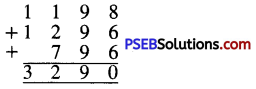Question 2.
Find the difference of 7693 and 4566.
Solution: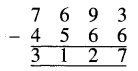Question 2.
The price of a fan is ₹ 11467 and the price of a cooler is ₹ 2215. How much total amount is required to buy both the things ?
Solution:
Cost price of the fan = ₹ 1467
Cost price of the cooler = ₹ 2275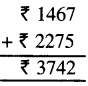Question 3.
Karan had ₹ 19080. He bought clothes worth ₹ 3705. How much amount was left with him ?
Solution:
The amount that Karan had with him = ₹ 9080
Amount spent on clothes = ₹ 3705
Amount left with him = ₹ 5375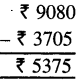Question 4.
In a library there are 3115 Punjabi books, 2876 Maths books and 976 English books. What is the total number of books in library ?
Solution:
Number of Punjabi books in the library = 3115
Number of Maths books in the library = 2876
Number of English books in the library = 976
The total number of books in library = 6967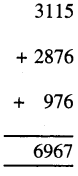Question 5.
The sum of two numbers is 9030. One number is 2141. Find the other number.
Solution:
Sum of two numbers = 9030
One number = 2141
Other number = 6889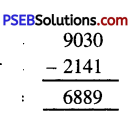Question 6.
What should be added in 7569 to get 9000 ?
Solution:
Sum = 9000
Number = – 7569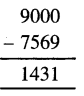= 1431

7. Find the number which is :

Question 1.
778 more than 3792
Solution:
Required number = 3792 + 778
= 4570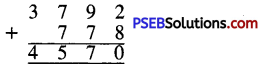Question 2.
515 less than 3777.
Solution:
Required number = 3777 – 515
= 3262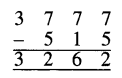Question 8.
The price of an almirah is ₹ 1595 and price of refrigerator is ₹ 6055 more than Almirah.
(a) Find the price of refrigerator
(b) Find the total price of almirah and refrigerator.
Solution:
Cost price of Almirah = ₹ 1595
(a) Cost price of refrigerator
= ₹ 1595 + ₹ 6055
= ₹ 7650.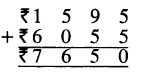(b) Total cost price of almirah and refrigerator
= ₹ 1595 + ₹ 7650
= ₹ 9245.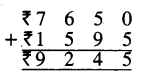Question 9.
Find the greatest and smallest 4 digit numbers by using digits 1, 4, 6 and 7 and also find the sum and difference of these numbers.
Solution:
The greatest 4 digit numbers = 7641
The smallest 4 digit numbers = 1467
Sum of these numbers = 9108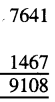Difference of these numbers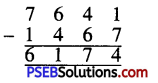Question 10.
Find the sum of the smallest 4 digit number and greatest 3 digit number.
Solution:
The smallest 4 digit number = 1000
The smallest 3 digit number = 999
Sum of these numbers = 1999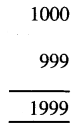Question 11.
Find the difference between place value of 8 and place value of 7 in the number 9874.
Solution:
Place value of 8 in the number
9874 = 8 × 100 = 800
Place value of 7 in the number
9874 = 7 × 10 = -70
Difference = 730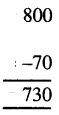Question 12.
Subtract 248 from smallest 4 digit number.
Solution:
The smallest 4 digit number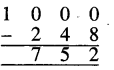Question 13.
Satnam had ₹ 765, his uncle gave him ₹ 250. Satnam gave ₹ 370 to his sister from the total amount. How much amount is left with him ?
Solution:
The amount that Satnam had with him = ₹ 765
The amount that his uncle has given to him = ₹ 250
The total amount that Satnam has now = ₹ 1015
The amount given by him to his sister = ₹ 370
The amount left with him = ₹ 645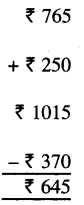Question 14.
Rozi had ₹ 1000. She bought a pair of shoes worth ₹ 150 and a suit worth ₹ 360. How much money was left with her ?
Solution:
The cost price of a pair of shoes = ₹ 150
The cost price of a pair of a suit = ₹ 360
The total cost price of both = ₹ 510
The total amount Rozi had with her = ₹ 1000
The amount spent by her = ₹ 510
The amount left with her = ₹ 490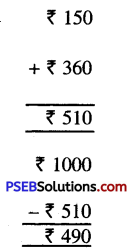Question 15.
Sandeep has ₹ 785 in his bank account. How much money should he deposit so that his total balance becomes ₹ 1000 ?
Solution:
The amount that should be in account = ₹ 1000
The amount he has in his account = ₹ 785
The amount that should be deposited = ₹ 215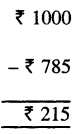Question 16.
The distance between Ferozepur to Chandigarh is 220 km. However distance between Ferozepur to Bathinda is 98 km. How much distance from Ferozepur to Chandigarh is more than that of Ferozepur to Bathinda ?
Solution:
The distance between Ferozepur and Chandigarh = 220 km
The distance between Ferozepur and Bathinda = 98 km
The distance from Ferozepur to Chandigarh is more than that of Ferozepur to Bathinda = 122 km.